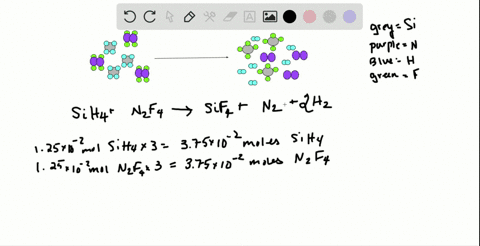Black Friday is Here! Start Your Numerade Subscription for 50% Off!Join Today### Write a balanced equation for the reaction depict…

05:20University of Maine
Problem 125

# When carbon-containing compounds are burned in a limited amount of air, some $\mathrm{CO}(g)$ as well as $\mathrm{CO}_{2}(g)$ is produced. A gaseous product mixture is 35.0 mass $\% \mathrm{CO}$ and 65.0 mass $\% \mathrm{CO}_{2} .$ What is the mass $\%$ of $\mathrm{C}$ in the mixture?

## Discussion

You must be signed in to discuss.

## Video Transcript

in a given reaction with oxygen, where we know the amount of carbon monoxide and carbon dioxide produced in terms of percentages. We confined the amount of carbon originally in the initial compound. So to do this we assume we have 100 grams of compound to call C X. And so if we assume that we have 100 grams, that means that we would have 65 grams of carbon dioxide and 35 grams with carbon monoxide. So to find how much of that 2% that's carbon. We change from grams to moles using the Moeller math and then we confined for every mole of Sio. Two. How many moles of carbon there are. And this is just based on the formula. And once we have moles, we confined grams again By using the molar mass of carbon, we repeat the same process for carbon monoxide, and this gives us the total amount of carbon and grams in 100 grams. So 65 grams of sio two one more and the molar mass of carbon is found by adding up the Mueller massive carbon in the periodic table and two times oxygen. It's 44 point no. One grams. And if we look at a molecule carbon dioxide, we can see that there's one carbon for every molecule of carbon dioxide. And so there's one mole of carbon for every mole of carbon tax. And then finally, to find her grams, we multiply by the molar mass carbon, which is 12 point of one grams, just 17 0.78 grams literally for carbon monoxide. Pretty grams of carbon monoxide. One more carbon monoxide has a molar mass of 28 quit 01 grams. And again there's one mole of Kurban and every mole of carbon monoxide. Changing two grams equals 15 0.1 grams through our total carbon 17.78 plus 15 +01 or 30 to 0.79 grams of carbon, and that's in 100 grams of compound, so the percentage carbon is 32.8%.